# Google Sheets: Get the Number of Words or Sentences in a Cell (Paragraph)Have you ever woken up in the middle of the night thinking – phawh! I’d really like to know how many words there are in a paragraph – Or stopped mid-chew on some arbitrary item carelessly left in the paddock by the boss and thought – geez! If there was only a way to count the number of sentences in a paragraph in a cell in Google Sheets!?

Well, you’re a bit weird, but I guess you are in the right place.

In this short tutorial, we will show you how to get the total count of words in a cell and the total count of sentences in a cell in Google Sheets. Hey, we will even throw in a how-to on getting the average sentence length in a paragraph, because we are nice like that – and we are a bit weird too.

We’ll give you the formulas for you to jump off on your own project straight away. We’ll also give you a detailed explanation of the formulas so you can figure out how it all works using our example.

## The example

In our example, we have a set of 4 paragraphs that we want to review: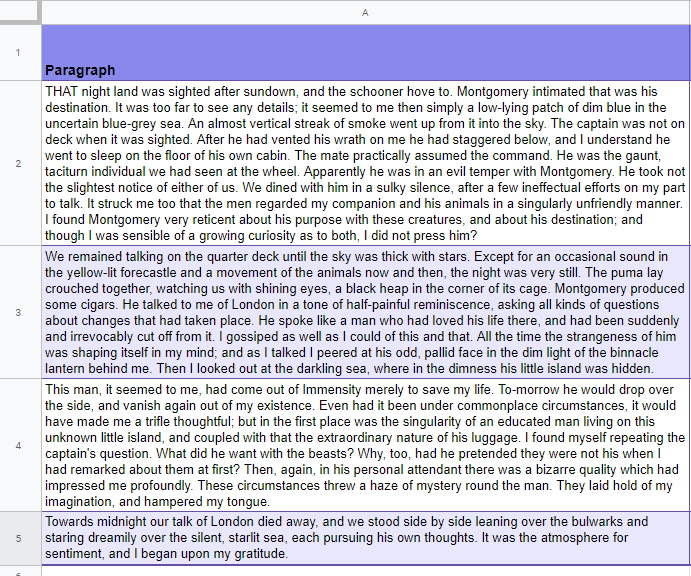We will want to extract the word count and the total number of sentences for each cell in column A. Then, we want to get the average word count for each sentence in each paragraph.

## Get the Total Number of Words in Each Cell (Paragraph) in Google Sheets

### The formula

=COUNTA(SPLIT(cell,” “))

The cell is the location of the paragraph. So in our example, our first cell with a paragraph is in A2. So our formula would look like this:

‘=COUNTA(SPLIT(A2,” “))### The explanation

Usually, the best way to look at a Google Sheet formula set is to start from the middle and work our way out.

#### Split(cell,” “)

The SPLIT function is used to separate text in a cell and put each separated block of text into its own cell to the right of the formula (and including the formula cell). The SPLIT function takes two necessary arguments:

SPLIT(text or cell location of text, delimiter)

In the text argument, you can either add the text directly to the SPLIT function or reference the text in a cell, like in our example (A2).

The delimiterarguments is the character or characters you want to use to split the text by.

Take a look at a simple example. Let’s say I have a cell A1 that contains the text:

Are you a dog? No,  I am a goat. You kwazy!

We can split out each word into its own cell by using an empty space in the delimiter argument. We do this by putting a space between two quotation marks, ” “.

Let’s see how this looks in Google Sheets:And here are the results:You can see now that each word is separated into its own cell. All we need to do now is count how many words there are in each cell.

#### countA(range)

COUNTA counts all the values in a set of data including text.

Let’s see how COUNTA works on its own. In cell A2, we will enter COUNTA and then select the range of the newly split words.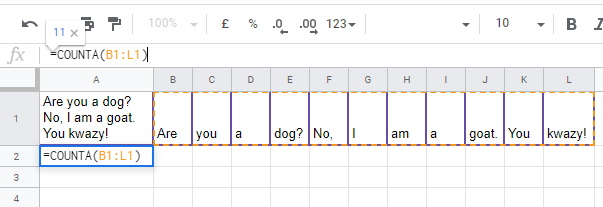You can see here, it returns 11.

So all we have to do is join our SPLIT and COUNTA into one formula:

=COUNTA(SPLIT(A1,” “))## Get the Total Number of Sentences in Each Cell (Paragraph) in Google Sheets

### The formula

=COUNTA(SPLIT(cell,“.!?”))

In our example, the cell reference would be A2. It would look like this:

=COUNTA(SPLIT(A2,“.!?”))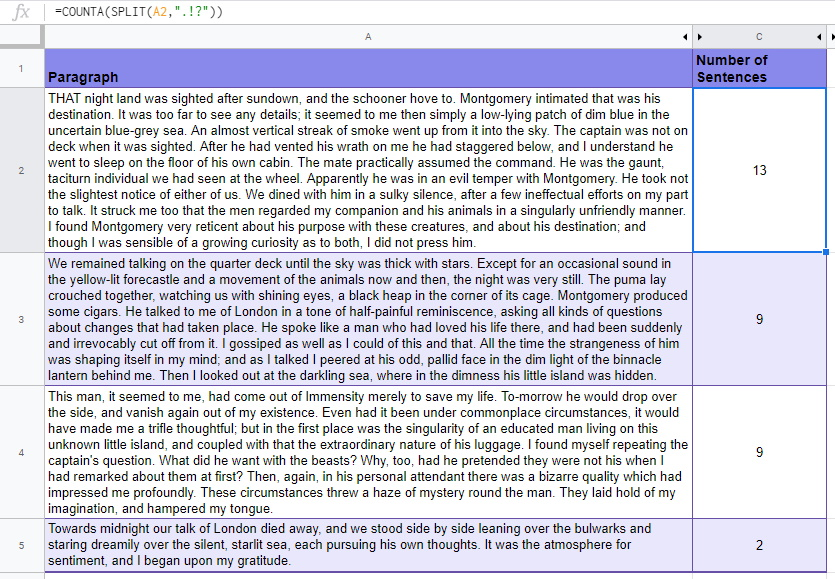### The explanation

We know that a sentence can conclude with a full stop (period) (.), question mark (?) or an exclamation mark (!).  In our delimiter argument, we added each sentence marker consecutively like this, “.!?”.

This way, the SPLIT function will check for each character “.”, “!” and “?” independently and spilt the text if it finds any of these. This is how SPLIT would look in our simple example:You can easily see here, we are going to get a count of 3 out of this when we add our COUNTA:

=COUNTA(SPLIT(A2,“.!?”))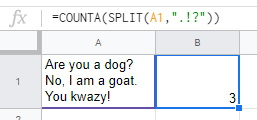## Get the average number of words in each sentence in Google Sheet

### The formula

There are two approaches here depending on your needs:

#### If you already have the total word and total sentence count in other cells

=ROUND(total number of words/total numbers or sentences,2)

In our example, the reference would look like this:

=ROUND(B2/C2,2)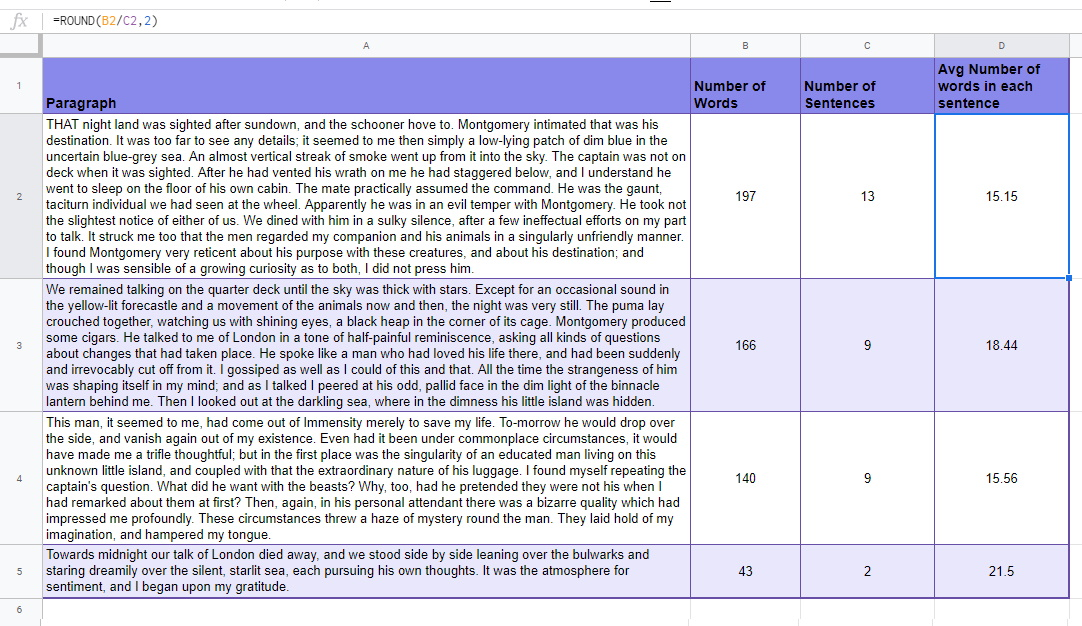#### If you JUST WANT TO REFERENCE THE AVERAGE WORD LENGTH OF SENTENCES IN EACH CELL

=ROUND(COUNTA(SPLIT(cell,” “)) /COUNTA(SPLIT(cell,“.!?”)),2)

And in our example:

=ROUND(COUNTA(SPLIT(A2,” “)) /COUNTA(SPLIT(A2,“.!?”)),2)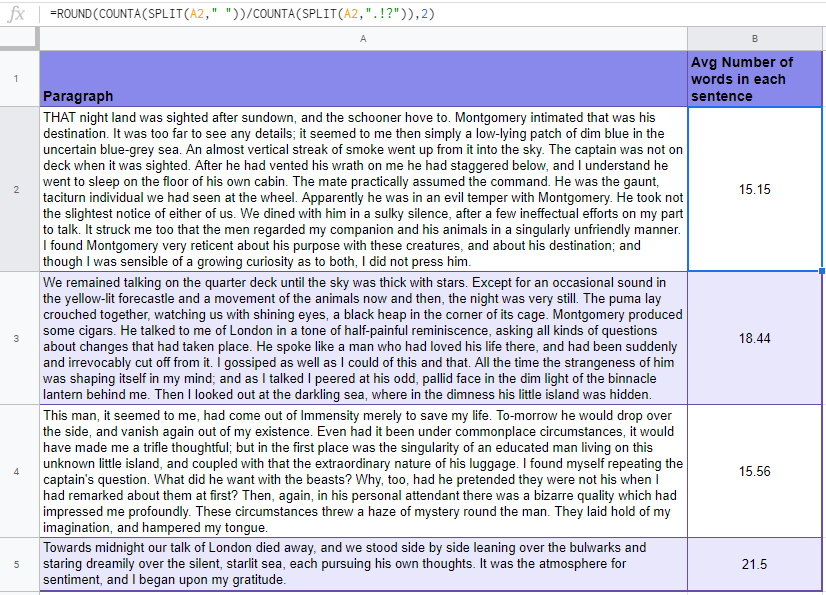### The explanation

#### Being average

To get the average number of words in each sentence in a cell, we divide the total number of words by the total number of sentences:

AVG Num Words = Word Count / Sentence Count

In our little example, it would look like this:#### round

We don’t have to see all those extra decimals we only want to see them to two decimal places. ROUND to the rescue.

ROUND take one primary argument, a number or a cell reference to a number. If left on its own, ROUND will round up or down to the nearest whole number. However, we can add an optional argument to determine how many decimal places ROUND should round to.

If we wanted to round 3.14159265359 to two decimal places, it would look like this:

=ROUND(3.14159265359, 2)

The result would be, 3.14.

Wrap ROUND around our average formula. It should now look like this:

=ROUND(B2/C2,2)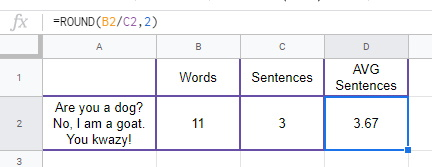### Building a Monster

Maybe the total number of words or the total number of sentences in a cell are not needed in the Google Sheet you are working on. What if we simply want the average number of words in each sentence?

All we need to do is to divide the number of words formula by the number of sentence formula:

=COUNTA(SPLIT(cell,” “))/COUNTA(SPLIT(cell,“.!?”))

In our little example it would look like this: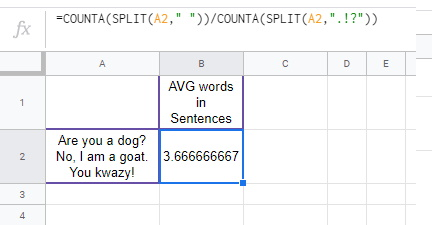Then we can wrap our ROUND function around this:

=ROUND(COUNTA(SPLIT(cell,” “))/ COUNTA(SPLIT(cell,“.!?”)),2)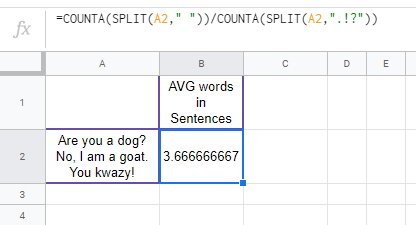Done! You have just created a delightful franken-formula.

## Conclusion

So, what have we used this for?

Well, it has been useful when getting the word count and paragraph count for student paragraph assignments. It also has come in handy for web scraping when trying to find endpoints or end of DIVs.

What will use this code on? I would love to hear in the comments below.

Here is a link to the example Google Sheet. Simply got to File >> Make a copy so that you have your very own version to play around with:

Number of  Words or Sentences in a Cell

Did you enjoy the tutorial? Want a solid step-by-step course to become a pro at Google Sheets? Check out my course, Google Sheets: Learn the Essentials with Three Detailed Projects. Sign up today.

Want a fast track to boost your Spreadsheet efficiency? Grab one of these handy Google Sheets Shortcuts mousepads that I created from my store.

1.Dennis G Daniels says: